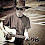## Tuesday, September 28, 2010

### Fibonacci rectangles and spirals

Make sure you read my Introduction to Fibonacci Numbers post before you read this...

Follow along with the diagram below.

Take a square with a side of 1 unit.

Add another square to the side (1 unit) of the original square.  Now you have a 2 x 1 rectangle.

Add another square to the larger (2 unit) side of this rectangle.  Now you have a 3 x 2 rectangle.

Add another square to the larger (3 unit) side of this rectangle.  Now you have a 5 x 3 rectangle.

Add another square to the larger (5 unit) side of this rectangle. Now you have an 8 x 5 rectangle.

Add another square to the larger (8 unit) side of this rectangle. Now you have a 13 x 8 rectangle.

As you can see, you can continue this indefinitely.  You've probably already noticed that the sides of the rectangles are succesive numbers from the Fibonacci sequence (1, 1, 2, 3, 5, 8, and 13 but future additions would yield 21, 34, 55, 89, etc.)

In my last post introducing the Fibonacci numbers, I mentioned that dividing successive Fibonacci numbers (Fn / Fn-1) approaches a constant (the higher the Fibonacci numbers, the more decimal place accuracy obtained for this constant).  The constant is called Phi (F).  The ratio of the two sides of these larger and larger rectangles similarly approach F which is why they are called Fibonacci rectangles.

Now, let's get interesting.  For each square that you've added, draw a 1/4 circular arc from corner to corner with the radius of the arc equal to the side of the square.  Continue in a clockwise direction from square to square and you'll find yourself drawing a nice spiral (here's an animation).

This is called a Fibonacci spiral for obvious reasons (technically, it approximates a Fibonacci spiral since these rectangle ratios approximate F).  There are objects in nature that look very much like Fibonacci spirals such as the chambered nautilus (Nautilus pompilius) shown below (sawn in half).

Here's a nice spiral galaxy (M74).

We see these types of curves in nature when an object's growth is proportional to its size.

#### 1 comment:

1.This surely must inscribe our mental processes as well, if we could see them :-D